##Advanced Graphics Study Guide

Advanced Graphics, Dr Neil Dodgson, University of Cambridge Computer Laboratory
Part II course, 2000

# 4C) Constructive Solid Geometry

Constructive solid geometry (CSG) essentially consists of Boolean set operations on closed primitives in 3D space. The three CSG operations are union, intersection and difference.

CSG is covered in FvDFH sections 12.7 and 15.10.3.

The following example illustrates the three CSG operations in use on simple three dimensional primitives.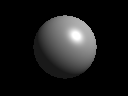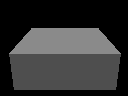The two primitives: a sphere and a box.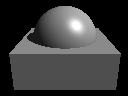The union of the two primitives.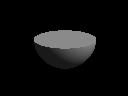The intersection of the two primitives.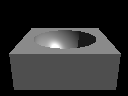The difference of the two primitives: box minus sphere.

The following example, based on FvDFH Plate III.2, shows an object for which CSG is (probably) the only sensible modelling technique. The object rendered in the right-hand image is constructed from the primitives shown in the left-hand image. It is mostly made out of cylinders, but you will recognise the extruded star from Part 4A.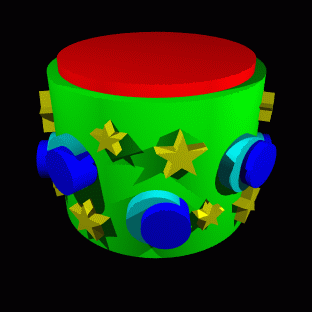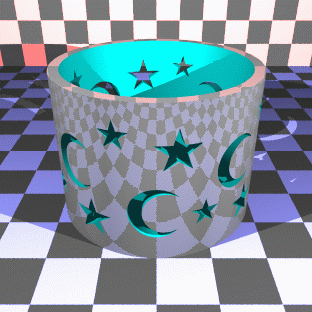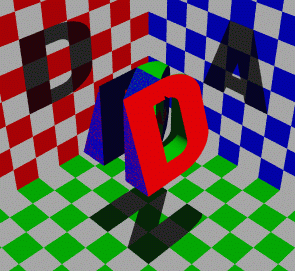At right, I tried the "Godel, Escher, Bach" treatment on my initials. Unfortunately the letters N, A, and D are not as amenable to this as the letters G, E, and B: notice that the shadow of the N has a slight curve at its top right, owing to the N's intersection with the curve on the D and the slope on the A. Various other arrangements of the three letters were tried, all of which gave more noticable artefacts than this. Each of the letters is a CSG object (the D, for example, is constructed from cylinders and boxes). The final effect is produced simply by intersecting the three letters.

 Exercises Work out how to represent a Lego technic brick as a CSG object. You may assume that you have box and cylinder primitives.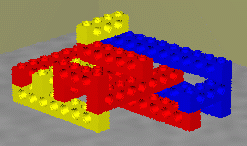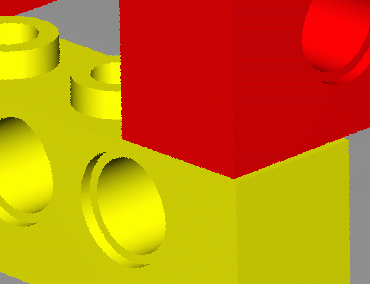[1998/7/12] Work out how to represent the following object using CSG. You may assume the following primitives: sphere, cylinder, cone, torus, box.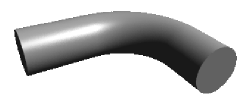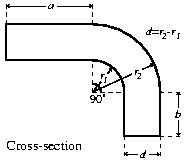[1999/9/4] (c) List the three ways of combining objects using constructive solid geometry (CSG). Describe how an object built using CSG can be represented using a binary tree. Given the intersection points of a ray with each primitive in the tree, explain how these points are passed up the tree by each type of combination node to produce a list of intersection points for the whole CSG object. [2000/9/4] (c) Describe how an object built using constructive solid geometry (CSG) can be represented using a binary tree. Given the intersection points of a ray with each primitive in the tree, show how to calculate the first intersection point of the ray with the entire CSG object.

Neil Dodgson | Advanced Graphics | Computer Laboratory

Source file: p4c.html
Page last updated on Thu Sep 14 16:58:08 BST 2000
by Neil Dodgson (nad@cl.cam.ac.uk)